Disclaimer: This is an example of a student written essay.
Click here for sample essays written by our professional writers.

Any scientific information contained within this essay should not be treated as fact, this content is to be used for educational purposes only and may contain factual inaccuracies or be out of date.

# Simulation of IV Bag Decanting

 ✅ Paper Type: Free Essay ✅ Subject: Sciences ✅ Wordcount: 3360 words ✅ Published: 4th Apr 2018

## Base Design and Equations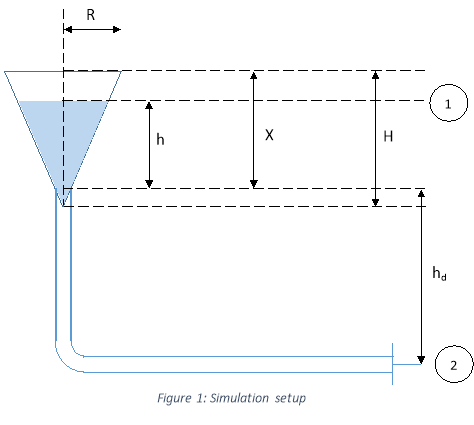The setup of the simulation is given below. The point at the top of the fluid in the IV bag is denoted with a subscript 1. The point at the exit of the catheter into the patient’s arm is denoted with a subscript 2. Assuming that the patient does not move his/her arm during the procedure, we can set point 2 as a static, whereas point 1 is moving dynamically as the bag empties.

Bernoulli’s Equation in the units of energy is given as: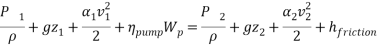Setting point 2 as the height of ground, excluding the pumping term and combining pressure terms into a pressure difference, but measuring P­1 and P2 in gauge pressure and assuming P1 to be atmospheric yields the following equation: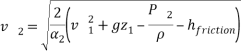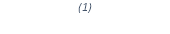Where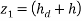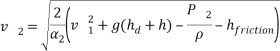Where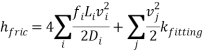For laminar flow (Re <= 2100):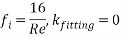And for turbulent (Re > 2100):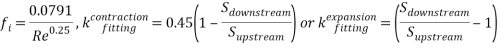## Mathematical Development

### Derivation of equations pertaining to the IV bag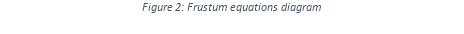What is needed first is a way to find the volume of fluid still within the bag when only knowing the height of the fluid in the bag. Taking the central axis of the bag as an independent variable and viewing the radius of the bag at that point as a dependent variable yields the following straight line:

Which has the equation: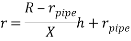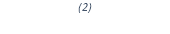And performing a solid of revolution about the h axis: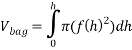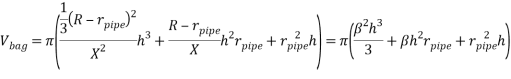It is know that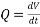and that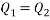by mass balance assuming no accumulation in the pipe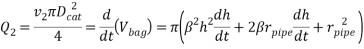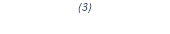But: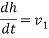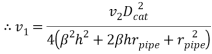Finally for the frustum IV bag: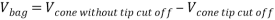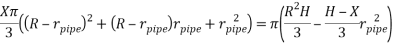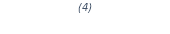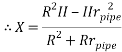### Friction terms development

Comparing the Reynold’s number in the pipe and the catheter: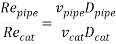But by mass balance and assuming no accumulation: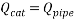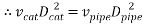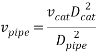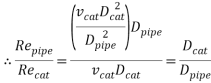Which is always less than one. Therefore if flow in the catheter is laminar, then flow in the pipe has to be laminar too.

This leaves 3 cases of flow in the system. The first is flow is laminar in the catheter and pipe. The second case is flow is turbulent in the catheter, but laminar in the pipe, or flow is turbulent for the catheter and pipe.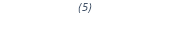For the first case where flow is laminar in both the catheter and the pipe: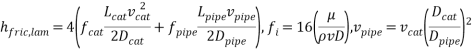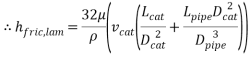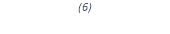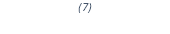If the flow is turbulent in the catheter, then the friction term is the sum of the friction from the pipe and the catheter: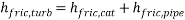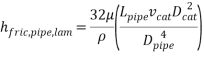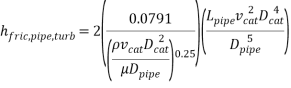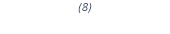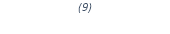## Simulation Program Development

### Solving velocity of fluid leaving the catheter for any fluid height h

The above Equations 1-9 are easily incorporated into matlab functions (Appendix A: Calculations functions).

The first problem that the program must solve is calculating the instantaneous velocity of fluid out of the catheter at any height of fluid in the IV bag.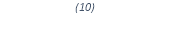First the flow in the catheter is assumed to be laminar (this is just a starting point for iterations). The correct velocity of the fluid leaving the catheter is the point here Equation 1 is stable.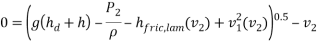Since the equation contains a square root, for the answer to be reasonable then there has to be a positive root. i.e: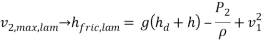Substituting equations 3 and 5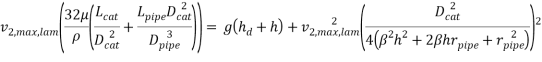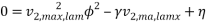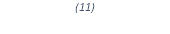Sinceis very small, it’s square may be neglected (checked with given simulation values it was on the order of 10-10)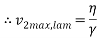The velocity that satisfies the stable solution of Equation 10 must therefore lie between 0 and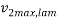for laminar flow speeds. This means that Equation 10 may be solved by bisection method, giving the instantaneous velocity out of the catheter for a certain height of fluid in the IV bag.

If the maximum and minimum velocities do not generate the proper conditions required for the bisection method (one function value must produce a number greater than zero, and the other must generate a number below zero to imply a root between the two numbers on a continuous function), then the flow velocity must be such that the flow is turbulent in the catheter.

If it is the case that flow must be turbulent in the catheter, then we can set an initial guess of v2 at the minimum velocity required to have turbulent flow in the catheter. Using this as an initial velocity, it can be iterated until a stable solution is found.

See Appendix B for this programming logic. Comments have been added to aid understanding.

### Fluid flow out of the IV bag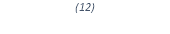In order to calculate how fluid flows out of the IV bag, we set the program to take small time elements and determine the velocity of the fluid flowing out of the catheter for a set fluid height. Since that time element is small, we assumed that the velocity did not change appreciably in that small element. Volumetric flow is the product of linear velocity of flow and cross-sectional surface area, and assuming no accumulation in the pipe for mass balance: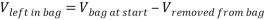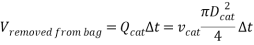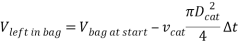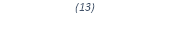Now we need to know what the height is of the bag for that volume, which is simply the real root that solves Equation 2.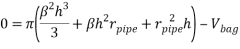This entire process is iterated for each time element until the bag empties or the fluid velocity leaving the catheter is zero.

This logic may be found in Appendix C.

### Concatenating Results

Finally a matrix is constructed that holds all data of the catheter fluid velocity, bag volume, fluid flow rate and height of fluid in the bag and is concatenated with a time vector. This resultant matrix now holds all of the data of the simulation, which is parsed to the primary class that is running the simulation to produce the graphs, and find numerical values for points on the graphs.

## Results of Simulation

The following data was fed into matlab:

P2 = 90.228/760*101325;

Lcat = 43.703/1000;

Dcat = 0.711/1000;

Lpipe = 1.383;

H = 22.8/100;

R = 0.3829*H;

hd = 1.377;

Dpipe = 3/1000;

rpipe = 0.5*Dpipe;

mu = 1.142/1000;

rho = 1017;

X = calcX(R,H,rpipe);

h0 = 0.8*X;

beta = (R-rpipe)/X;

The simulation was run using the following command to retrieve the results matrix:

VhvQt = generateVhvtQMatrix(rho,Dcat,mu,Lpipe,Lcat,Dpipe,beta,h0,rpipe,9.81,hd,P2);

Solution graphs were plotted as follows:

plot(VhvQt(5,:),VhvQt(2,:));

xlabel(‘Time in seconds’);

ylabel(‘Height of fluid in bag in meters’);

Bag volume is row 1, height of bag is row 2, velocity of fluid in the catheter is row 3, flowrate is row 4 and row 5 is the time vector. To find the total time taken for the bag to empty, the command was used:

VhvQt(5,end)

= 3122

Similar commands were used to find exact datapoints at any period in time.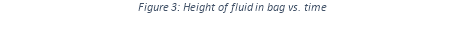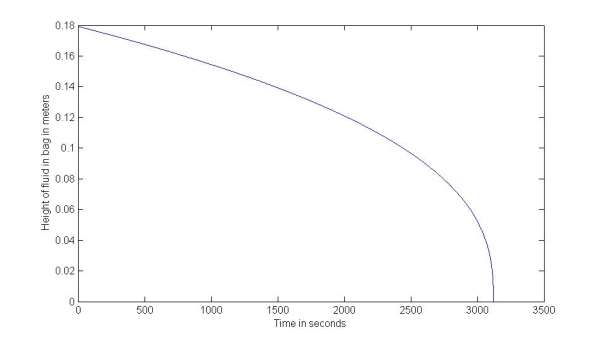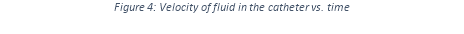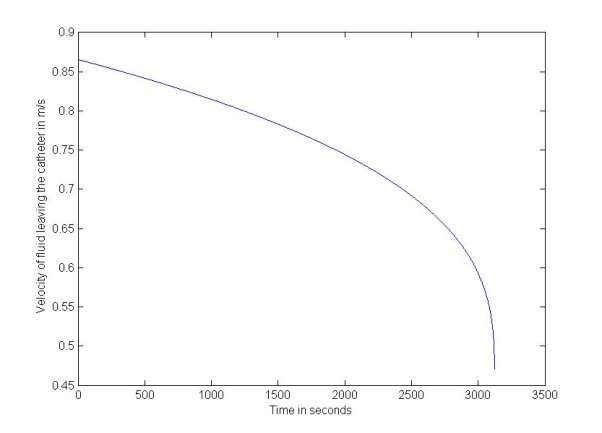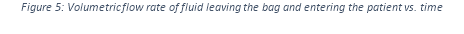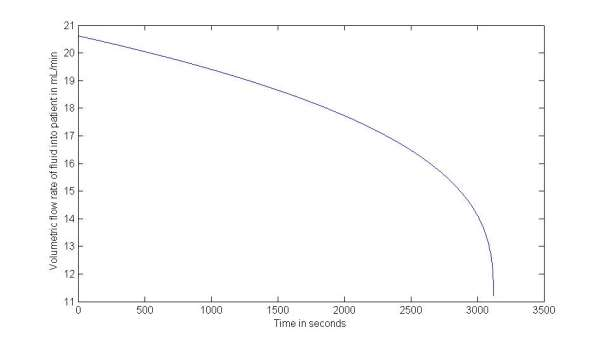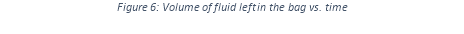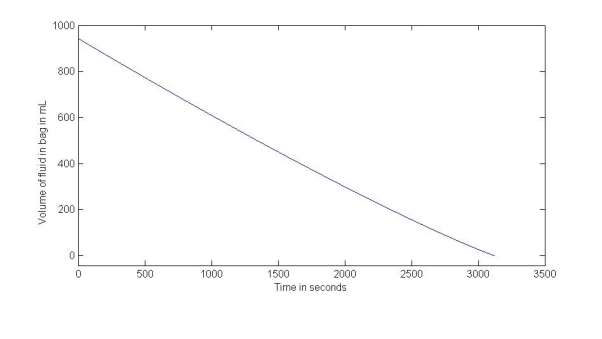## Discussion of Simulation Results

The values of the viscosity and density of the 5% w/v glucose solution – more commonly known as D5W, Dextrose 5 Water or Intravenous Sugar Solution, were looked up from Wolfram Alpha’s material database, and had the values of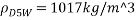and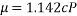. (Wolfram Alpha)

The purpose of the simulation was to calculate and plot the graphs as well as answer how long it takes for the bag to drain.

It took 3122 seconds for the bag to drain, or just over 52 minutes. At the last second of the simulation, the height of fluid in the bag was less than 4 millimetres, which corresponds to a volume of 0.045 mL. Figures 3-5 all show a negative exponential function, which is to be expected since the flow rate is a function of the head pressure, which is a function of the conical shape of the bag. Initially, the high flow rate that corresponds with the higher head of fluid does not change very much, as the level of fluid in the cone does not drop significantly quickly. However, after about 2500 seconds (80% through the time of the simulation), the velocity of fluid leaving the catheter begins to change.

Find Out How UKEssays.com Can Help You!

Our academic experts are ready and waiting to assist with any writing project you may have. From simple essay plans, through to full dissertations, you can guarantee we have a service perfectly matched to your needs.

While this change is clearly not linear, it is not really significant when compared to the absolute changes in values. From the start of the simulation until 80% through the simulation (2500 seconds), the velocity of fluid in the catheter only changes from 0.8655 to 0.6922 m/s, or 20.02%. It is only in the last period of time just before the bag empties is there a significant change in the velocity of fluid entering the patient, with the final velocity being 0.4707 m/s just as the bag empties, which is itself only a change of 45.62%.

This phenomenon is most apparent in Figure 6, where it can be seen that the volume of fluid left in the bag appears to decant at an almost linear rate (differential of volume/time is flow rate). Only just after 2500 seconds does the steepness of the graph very slightly begin to change upward indicated a slow in the flow rate of fluid leaving the bag.

This phenomenon does, however, make sense. The height that the bag is lifted above the catheter is hd = 1.377 meters. The height of fluid in the bag at the start of the procedure is h0 = 0.1793m, or only 13.20% of hd. The means that the driving force behind the IV procedure (which is the height above the IV bag is placed above the catheter in the patient’s arm) that creates the pressure difference to overcome the venous pressure of blood in the patient’s veins only changes by 13.2%, and remains changed by only 20.02 % during the initial 80% of the procedure – in other words, it doesn’t change by much, and therefore we expect that the flow rate won’t change very much until very close to the end of the procedure, which is what we see in Figure 3.

In real world application, this means that in general the flow rate of IV solution to the patient is mostly a function of how high above the patient the IV bag is placed, and not necessarily how empty or full the bag its self is. In fact, general practise used by doctors is simply to place the IV bag above the heart level of the patient (Dr. Chen-Maynard, 1999). In application, a desired flow rate of IV fluid into the patient may controlled by lowering or lifting the IV bag a certain height above the patient.

## Nomenclature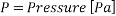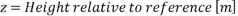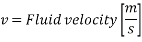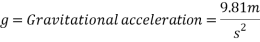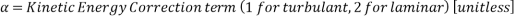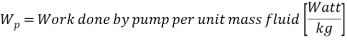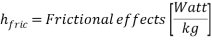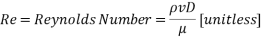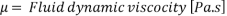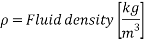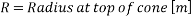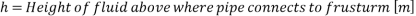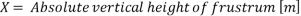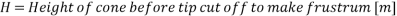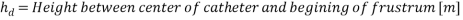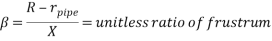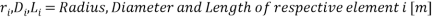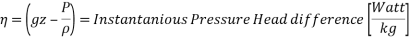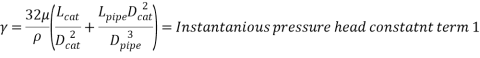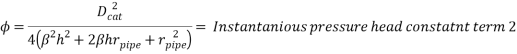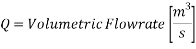## References

Dr. Chen-Maynard, P. R. (1999). Calculating Parenteral Feedings. California: California Department of Health Science and Human Ecology.

Wolfram Alpha. (n.d.). Comprehensive Material Data Sheet for D5W. Retrieved April 28, 2014, from http://www.wolframalpha.com/input/?i=5%+(w/v)+glucose

## Cite This Work

To export a reference to this article please select a referencing stye below:

View all

## DMCA / Removal Request

If you are the original writer of this essay and no longer wish to have your work published on UKEssays.com then please:

Related Services

Related Lectures

Study for free with our range of university lectures!Freelance Writing Jobs

Looking for a flexible role?
Do you have a 2:1 degree or higher?

Study Resources

Free resources to assist you with your university studies!